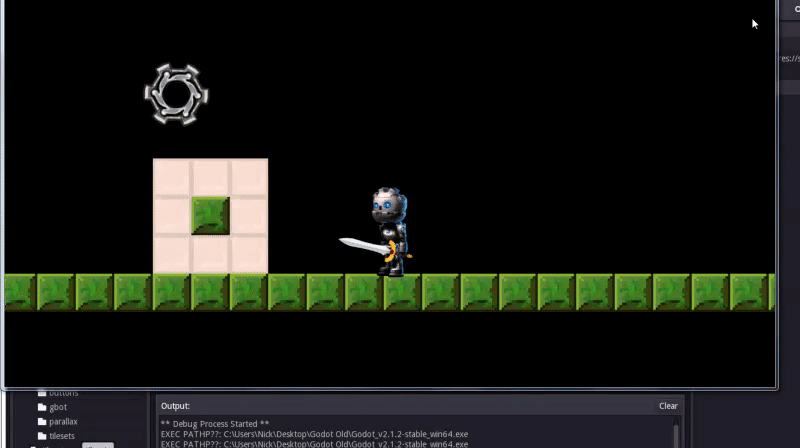Man, I am so close on getting this movement down! Someone helped me fix a small issue I had here.

But now, the jitter is back but it can be removed by interpolation. I know that, but am horrible at Math and trying to figure out how to get a dynamic delta.

Code:

``````var _velocity = Vector2(0,0)
var SPEED = 1000
func _process(delta):
if new_pos.x:
var my_pos = get_pos()
var vector = new_pos - my_pos
var length = floor(vector.length())
var direction = vector.normalized()
if length < 2: #2px, experiment with this value
if length < 0.5:
_velocity = Vector2(0,0) # full stop.
else:
_velocity = vector * SPEED * delta # sloooooowly
else:
_velocity = vector * SPEED * delta

func _integrate_forces(state):
# if the path has more than one point
if new_pos.x:
state.set_linear_velocity(_velocity)
``````

And, if server is sending positional updates to `new_pos` 15 times a second, I get:So, what I am doing is basically sending a `Vector2` of x, and y positions to my server and relaying it back to the other player at 15 times per second. (`new_pos`) is getting its value updated from data received from the server.

Now, my question is to remove the jitter I can either do 60 times per second interval (which would rape my servers), or simply interpolate the `time` difference between packets and create a dynamic `delta`. I'm just not sure how to do that.

I used `OS.get_ticks_msec()` when I send the msg to the server and put it in a variable called `last_message_sent`, and I did the same for when I receive a message from the server. That variable is called `last_message_received`.

I am so close, but am kind of stuck on how to get `delta`'s value to be dynamic so the jitter is interpolated, any help is greatly appreciated! Thanks in advance!!

in Engine